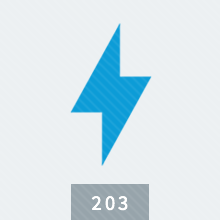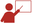# Transformers and AC Circuits

The Transformers and AC Circuits online electrical course covers differences between DC and AC circuits. Explains AC sine wave, using vectors to solve AC problems, calculating impedance in circuits having inductance, capacitance, and resistance, AC power relationships in single-phase and three-phase circuits, and principles of transformer maintenance. Transformers and AC Circuits is available in online technical training and course manual formats.

TPC Training is authorized by IACET to offer 1.0 CEUs for this program.

Review a full training course list for Electrical and Instrumentation TechnicianLooking for Live training on Electrical Systems?

#### Select Format

• Online Course & Test \$85.00
• Course Manual \$71.00
• Course Manual (Spanish) \$71.00

TPC Training is authorized by IACET to offer 0.5 CEUs for Transformers and AC Circuits online training.

#### Lesson 1 - Principles of Alternating Current

##### Topics:

AC and DC electricity; AC waveform; Peak-to-peak, average, effective values; Energy storage; Faraday's Law; Basic circuit concepts

##### Learning Objectives:
– State of definition of a waveform.
– Demonstrate how to calculate the frequency of an alternator's output.
– Explain how to calculate an effective value.
– Name the kinds of values that must be used when applying the dc rules and laws to ac circuits.

#### Lesson 2 - Mathematics in AC Circuits

##### Topics:

Potential difference; Angles and degrees; Vectors applied to AC circuits; Graphic and math solutions; Calculating instantaneous values

##### Learning Objectives:
– Describe a triangle.
– State the definition of a vector.
– Identify the vector representing resistance in a vector diagram.
– Demonstrate how to calculate the total impedance in an ac circuit.

#### Lesson 3 - Inductance and Inductive Reactance

##### Topics:

Factors affecting inductance; CEMF; Inductive reactance and time delay; Phase angles; Impedance; Mutual induction; Inductors

##### Learning Objectives:
– Name the property of a coil that makes it resist changes in current.
– List the factors that determine inductance in a coil.
– State the definition of counter electromotive force.
– Demonstrate how to convert a frequency in Hz to a frequency in radians per second.

#### Lesson 4 - Capacitance and Capacitive Reactance

##### Topics:

How a capacitor works; Factors controlling capacitance; Kinds of capacitors; Time constants; Capacitive reactance; Phase angle

##### Learning Objectives:
– Name the parts of a capacitor.
– List the factors that affect the amount of charge stored in a capacitor at a given potential difference.
– Demonstrate how to install a multisection electrolytic capacitor.
– State the definition of capacitive reactance.

#### Lesson 5 - Impedance

##### Topics:

Impedance in series circuits; Phase angles; Resonance in series circuits; Impedance in parallel circuits

##### Learning Objectives:
– State the definition of impedance
– Explain how to calculate the impedance in a series ac circuit.
– Demonstrate how to find the value of a phase angle for a circuit.
– Explain how to calculate the impedance in a parallel circuit.

#### Lesson 6 - Power and Energy in AC Circuits

##### Topics:

Work and energy; Power in resistive, inductive, and capacitive circuits; Power factor correction; Power capacitors; Capacitor installation

##### Learning Objectives:
– State the definition of power.
– Demonstrate how to calculate power in an inductive circuit.
– State the reason why capacitors are added to circuits to increase the power factor.
– Explain how to install capacitors correctly.

#### Lesson 7 - Three-Phase Circuits

##### Topics:

Three-phase alternators; Y- and delta-connected alternators; Power in three-phase circuits; Load connections; Measuring power

##### Learning Objectives:
– List the main advantages of the three-phase ac system.
– State the definition of phase sequence.
– Demonstrate how to calculate the RMS power in a single-phase circuit.
– Explain how to measure the total power consumed by the load in a three-phase circuit.

#### Lesson 8 - Principles of Transformers

##### Topics:

Magnetic field; No-load operation; Transformer construction, losses, and efficiency; Autotransformers; Instrument transformers

##### Learning Objectives:
– Explain the difference between the primary winding and the secondary winding in a transformer.
– Explain how the windings are positioned in a core-type transformer.
– List the kinds of losses that occur in transformers.
– State the definition of a current transformer.
– List the functions of an instrument transformer.

#### Lesson 9 - Transformer Applications

##### Topics:

Transformer designation, insulation, cooling, and polarity; Single- and three-phase transformer connections; Installing transformers

##### Learning Objectives:
– Name general kinds of transformers.
– List the temperature limits for each class of transformer insulation.
– Explain how oil-immersed transformers are cooled.
– Name the common methods of connecting three single-phase transformers for three-phase operation.
– Explain how to select the correct location for a transformer.

#### Lesson 10 - Maintaining Transformers

##### Topics:

Preventive maintenance; Inspection; Transformer liquids; Transformer failure; Testing; Disassembly and inspection

##### Learning Objectives:
– Explain what to look for during an inspection of sealed transformers.
– List problems that are indicated by an increase in transformer operating temperature.
– Demonstrate how to perform a breakdown test.
– Explain how to locate the exact point of a leak in a welded joint below the liquid level.
– List the steps in inspecting a transformer when a winding fails.